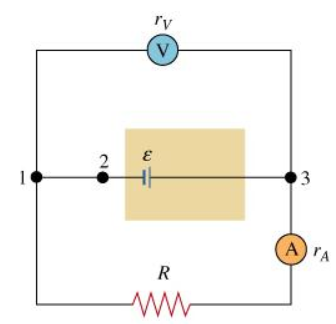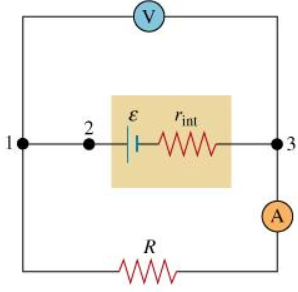###### FREE Expert Solution

Part A

Kirchoff's loop rule:

$\overline{){\mathbf{\Sigma }}{\mathbf{V}}{\mathbf{=}}{\mathbf{0}}}$

V = ε - IR

There is no resistance across points 1 and 3. Due to the very large resistance across the voltmeter, V, no current flows in the voltmeter.

92% (305 ratings)###### Problem Details

Consider the circuit shown. (Intro 1 figure) All wires are considered ideal; that is, they have zero resistance. We will assume for now that all other elements of the circuit are ideal, too: The value of resistance is a constant, the internal resistances of the battery and the ammeter are zero, and the internal resistance of the voltmeter is infinitely large.

Intro 1:Intro 2:Part A

What is the reading V of the voltmeter?

Part B

The voltmeter, as can be seen in the figure, is connected to points 1 and 3. What are the respective voltage differences between points 1 and 2 and between points 2 and 3?

a) 0; ℰ

b) 0; -ℰ

c) ℰ; 0

d) ℰ;ℰ

Part C

What is the reading I of the ammeter?

Now assume that the battery has a nonzero internal resistance r (but the voltmeter and the ammeter remain ideal). (Intro 2 figure)

Part D

What is the reading of the ammeter now?

Part E

What is the reading of the voltmeter now?